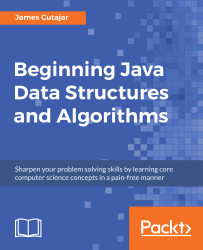•#### Beginning Java Data Structures and Algorithms#### Overview of this book

Learning about data structures and algorithms gives you a better insight on how to solve common programming problems. Most of the problems faced everyday by programmers have been solved, tried, and tested. By knowing how these solutions work, you can ensure that you choose the right tool when you face these problems. This book teaches you tools that you can use to build efficient applications. It starts with an introduction to algorithms and big O notation, later explains bubble, merge, quicksort, and other popular programming patterns. You’ll also learn about data structures such as binary trees, hash tables, and graphs. The book progresses to advanced concepts, such as algorithm design paradigms and graph theory. By the end of the book, you will know how to correctly implement common algorithms and data structures within your applications.
Title PagePackt UpsellContributorsPrefaceFree Chapter
Algorithms and ComplexitiesSorting Algorithms and Fundamental Data StructuresHash Tables and Binary Search TreesString Matching AlgorithmsGraphs, Prime Numbers, and Complexity ClassesOther Books You May EnjoyIndex## Chapter 4. Algorithm Design Paradigms

In the previous chapter, we learned about hash tables and binary search trees. In this chapter, we will explore algorithm design paradigms. These design patterns can be seen as the generic methods or approaches that motivate the design of a class of algorithms.

Just as an algorithm is a higher abstraction than a computer program, an algorithm design paradigm is an abstraction higher than an algorithm. The choice of an algorithm paradigm is an important one when designing an algorithm.

This chapter will focus on the following three algorithm paradigms:

• Greedy
• Divide and conquer
• Dynamic programming

By becoming familiar with these higher abstractions, you can make more informed decisions when designing algorithms.

### Note

In a previous chapter, we have come across the merge sort and quick sort algorithms, which are examples of the divide and conquer paradigm. As the name suggests, both of these algorithms divide the input into smaller parts, which are then solved...